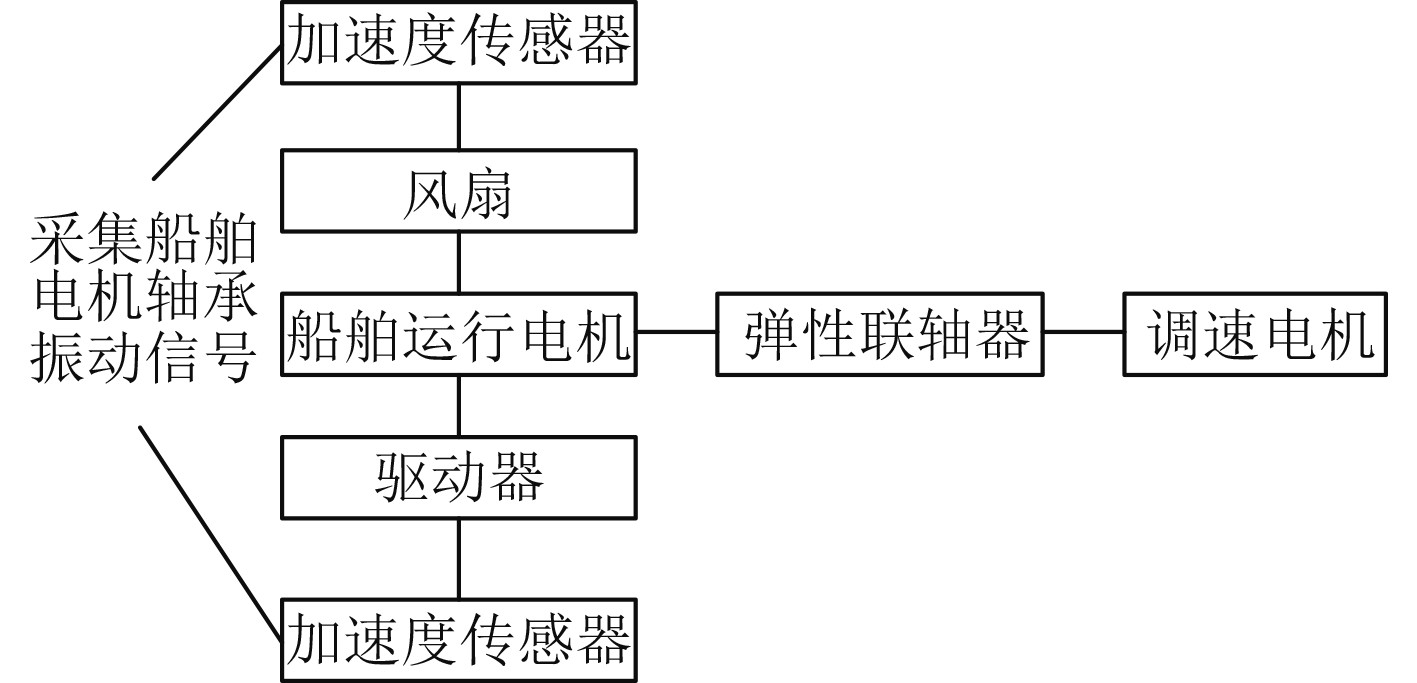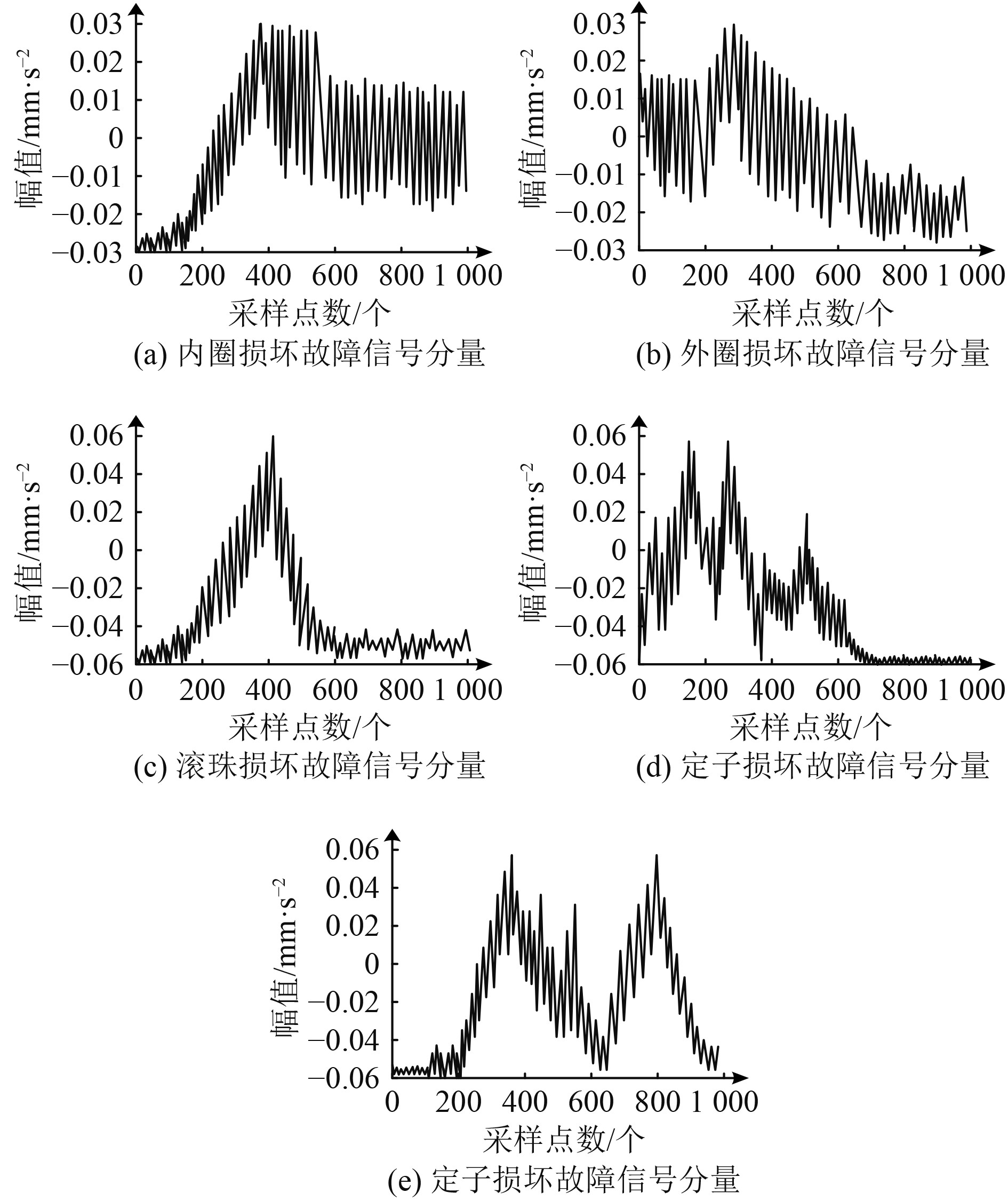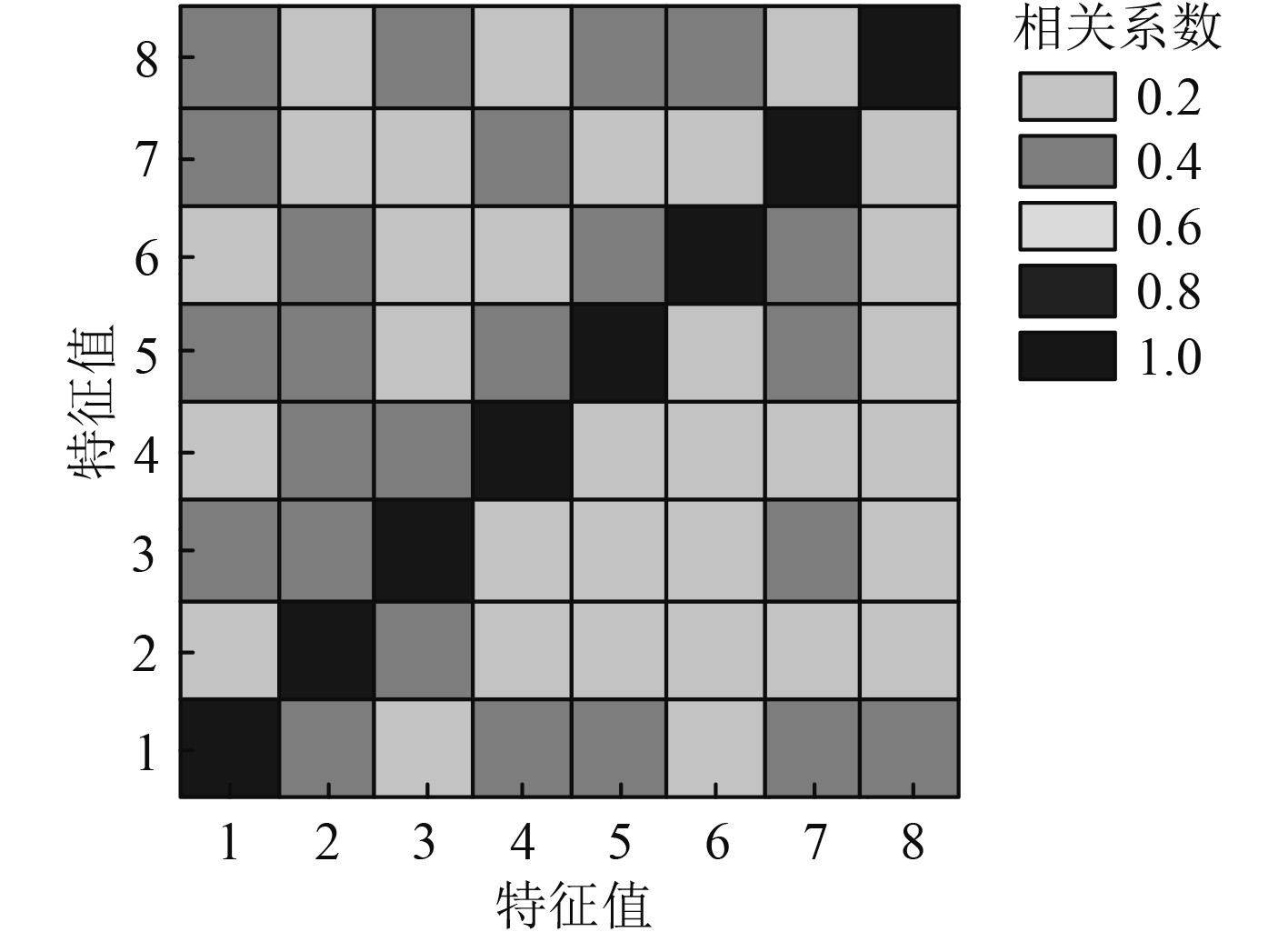﻿ 改进MSEA-CNN的船舶电机轴承故障诊断方法
 舰船科学技术2022, Vol. 44Issue (14): 119-122    DOI: 10.3404/j.issn.1672-7649.2022.14.025PDF

1. 交通运输部南海航海保障中心广州航标处，广东 广州 510000;
2. 交通运输部水运科学研究院，北京 100088

Research on fault diagnosis method of marine motor bearing based on improved MSEA-CNN
LI Zhong1, WANG Wen-kai2, WANG Peng-fei1
1. Guangzhou Aids to Navigation Department, NGCS, MOT, Guangzhou 510000, China;
2. China Waterborne Transportation Research Institute, Beijing 100088, China
Abstract: Because there are many types of ship motor bearing signals, and most of them are useless signals, which reduces the correlation between fault signal components, the fault diagnosis method of Ship Motor Bearing Based on msea-cnn is studied and improved. Using adaptive linear neural network, a filter for removing non bearing fault components is designed, and the fault signal components are removed from the bearing vibration signal to extract the bearing fault signal components. Through the generative countermeasure neural network, the sample labels of each fault signal component are obtained to realize the classification of fault signal components. The sample label and fault signal component are fused to obtain the fault diagnosis training set. A fault diagnosis model is established by using attention and multi-scale convolution neural network. Experiments show that this method can effectively extract fault signal components. The correlation coefficient between fault signal component features extracted by this method is low, which shows that this method has better feature extraction effect.
Key words: countermeasure neural network     marine motor     bearing failure     wave filter     fault signal component     diagnostic model
0 引　言

1 船舶电机轴承故障诊断 1.1 故障信号分量提取

 ${\boldsymbol{P}} = \left[ \begin{array}{c} p\left( k \right) \\ p\left( {k - 1} \right) \\ \vdots \\ p\left( {k - n} \right) \\ \end{array} \right],\;t = p\left( k \right)。$ (1)

 $F\left( X \right) = E\left[ {t - y{{\left( n \right)}^2}} \right]\eta = E\left[ {{{\left( {t - {X^{\rm{T}}}\varpi } \right)}^2}} \right]\eta。$ (2)

 $\begin{gathered} F\left( X \right) = E\left[ {{t^2} - 2t{X^{\rm{T}}}\varpi {\varpi ^{\rm{T}}}X} \right]\eta =\\ E\left[ {{t^2}} \right]\eta - 2{X^{\rm{T}}}E\left[ {t\varpi } \right]\eta + {X^{\rm{T}}}E\left[ {\varpi {\varpi ^{\rm{T}}}} \right]X\eta 。\\ \end{gathered}$ (3)

 $\begin{gathered} \nabla F\left( X \right) = 0 \Rightarrow \nabla \left( {C - 2{X^{\rm{T}}}h + {X^{\rm{T}}}RX} \right)\eta = 0 \\ \Rightarrow - 2h\eta + 2RX\eta 。\\ \end{gathered}$ (4)

 $X = \frac{h}{R}。$ (5)
1.2 基于对抗卷积神经网络的船舶电机轴承故障诊断

1.2.1 轴承故障信号分量分类

 $\begin{split}&L = - \left\{ \begin{gathered} {{E'}_{e\left( n \right){\text{~}}v\left( {e\left( n \right)} \right)}}\log \left[ {1 - v'\left( {z = K + 1\left| {e\left( n \right)} \right.} \right)} \right] \\ + {{E'}_{e\left( n \right){\text{~}}G}}\log \left[ {v'\left( {z = K + 1\left| {e\left( n \right)} \right.} \right)} \right] \end{gathered} \right\} = \\ &- \left( {{{E'}_D} + {{E'}_G}} \right)。\end{split}$ (6)

1.2.2 船舶电机轴承故障诊断模型

MSEA-CNN网络内主要包含注意力特征加权、多尺度特征提取与故障诊断三部分。第一部分通过注意力机制自动获取，各标签下船舶电机轴承故障信号分量内特征的重要程度，按照该重要程度屏蔽无用特征，凸显有用特征，令MSEA-CNN网络在输入各标签下船舶电机轴承故障信号分量特征内，得到存在较强判别性的信息。

 $\omega = \sigma \left( {f\left( {A'} \right)\zeta } \right) = \left[ {{\omega _1},{\omega _2}, \cdots ,{\omega _l}} \right]。$ (7)

 $M = \delta \left( {f'\left( {A'} \right)\zeta } \right) = \left[ {{m_1},{m_2}, \cdots ,{m_l}} \right]。$ (8)

 $\hat A\left( i \right) = \frac{{\lambda \displaystyle\sum\limits_{j = 1}^l {{{A'}_{i,j}}} }}{l}。$ (9)

2 实验分析图 1 船舶电机轴承实验平台 Fig. 1 Marine motor bearing test platform图 2 各故障信号分量提取结果 Fig. 2 Extraction results of each fault signal component图 3 轴承故障信号分量特征提取效果 Fig. 3 Feature extraction effect of bearing fault signal components表 1 船舶电机轴承故障诊断结果 Tab.1 Bearing fault diagnosis results of marine motor

3 结　语

  张仲懿, 姚玉南, 梁言. 基于贝叶斯网络的船舶发电机运行过热故障分析[J]. 武汉理工大学学报(交通科学与工程版), 2019, 43(2): 311-315.  杨晓珍, 周继续, 邓举明. 基于改进贝叶斯分类的电机轴承故障诊断系统研究[J]. 机床与液压, 2020, 48(20): 172-175. DOI:10.3969/j.issn.1001-3881.2020.20.040  姜磊, 朱明斯, 李飞龙. 基于AMD和小波阈值去噪的电机轴承故障诊断[J]. 机械设计与制造, 2021(9): 47-50. DOI:10.3969/j.issn.1001-3997.2021.09.011  张习习, 顾幸生. 基于集成学习概率神经网络的电机轴承故障诊断[J]. 华东理工大学学报(自然科学版), 2020, 46(1): 68-76. DOI:10.14135/j.cnki.1006-3080.20181206001